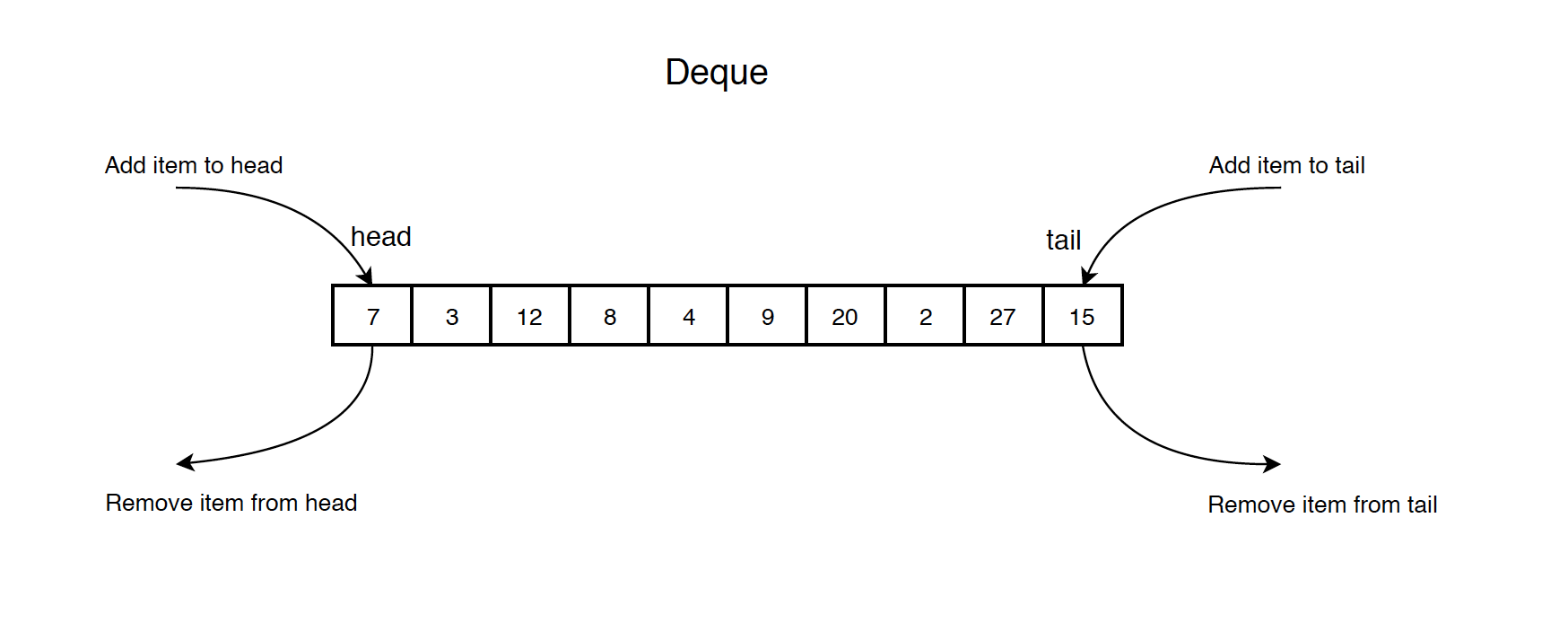# 1114. Data Structure - DequeDeque

Implement deque with linked list and circular array.

## 1. Deque

### 1.1 Real-life Example

Undo and Redo function in text editor.

### 1.2 Deque in Programming Terms

A double-ended queue (abbreviated to deque) is an abstract data type that generalizes a queue, for which elements can be added to or removed from either the front (head) or back (tail). It has four principal operations:

• addFirst: add an element to the head
• addLast: add an element to the tail
• removeFirst: remove the first element
• removeLast: remove the last element### 1.3 Common Operations on Queue

• removeFirst(): Pull the first item out of the list.
• removeLast(): Pull the last item out of the list.
• peekFirst(): Return the first item of the deque.
• peekLast(): Return the last item of the deque.
• isEmpty(): Return true if the deque is empty.

### 1.4 Time Complexity

• addFirst: $O(1)$
• addLast: $O(1)$
• removeFirst: $O(1)$
• removeLast: $O(1)$
• peekFirst: $O(1)$
• peekLast: $O(1)$

## 2. Implementation

Define Node.

public class ListNode {
public int val;
public ListNode prev;
public ListNode next;
public ListNode(int val) {
this.val = val;
this.prev = null;
this.next = null;
}
}


Implement Deque.

public class LinkedListDeque {
private ListNode head; // the first node
private ListNode tail; // the last node

tail = null;
}

} else {
}
}

// Remove the head from the list and return its value
public int removeFirst() throws Exception {
throw new Exception();
}
} else {
tail = null;
}
return value;
}

// Get the value of the head
public int peekFirst() throws Exception {
throw new Exception();
}
}

// Add item to the tail of the list
if (tail == null) {
tail = new ListNode(value);
} else {
tail.next = new ListNode(value);
tail.next.prev = tail;
tail = tail.next;
}
}

// Remove the tail from the list and return its value
public int removeLast() throws Exception {
if (tail == null) {
throw new Exception();
}
int value = tail.val;
tail = tail.prev;
if (tail != null) {
tail.next = null;
} else {
}
return value;
}

// Get the value of the tail
public int peekLast() throws Exception {
if (tail == null) {
throw new Exception();
}
return tail.val;
}

// Return whether the deque is empty
public boolean isEmpty() {
return head == null || tail == null;
}
}


### 2.2 Using Circular Array

Use MOD to get the new position.

public class CircularArrayDeque {
private int head; // the first node in deque, not the first item in array
private int tail; // the last node in deque, not the first item in array
private int[] arr;
private int size;

public CircularArrayDeque(int capacity) {
arr = new int[capacity];
tail = 0;
size = 0;
}

// check if deque is full
if (isFull()) {
return;
}

}
size += 1;
}

// Remove the first item from the deque and return its value
public int removeFirst() throws Exception {
if (isEmpty()) {
throw new Exception("Circular Array Deque is empty when dequeue!");
}
size -= 1;
return value;
}

// Get the first item
public int peekFirst() throws Exception {
if (isEmpty()) {
throw new Exception("Circular Array Deque is empty when peek!");
}
}

// Add item to the end of the deque
// check if deque is full
if (isFull()) {
return;
}
tail = (head + size) % arr.length;
arr[tail] = value;
size += 1;
}

// Remove the last item from the deque and return its value
public int removeLast() throws Exception {
if (isEmpty()) {
throw new Exception("Circular Array Deque is empty when dequeue!");
}

int value = arr[tail];
tail = tail - 1;
if (tail < 0) {
tail = arr.length - 1;
}
size -= 1;
return value;
}

// Get the last item
public int peekLast() throws Exception {
if (isEmpty()) {
throw new Exception("Circular Array Deque is empty when peek!");
}
return arr[tail];
}

// Return whether the queue is full
public boolean isFull() {
return size == arr.length;
}

// Return whether the queue is empty
public boolean isEmpty() {
return size == 0;
}
}